# Introduction:

We see that we have a lot of problems in the life of Computer Science. Many of the problems are solved, or we can say that algorithms are made to solve the problem. But yes, it is not the end. People are working to solve the problem efficiently, like making an exponential-time solvable problem to a polynomial-time solvable problem.

People in the research field are always optimistic depending on the progress. If for some reason they are unable to reach their goal, they would like to preserve their work, which saves them hardship and makes it easier for others to take up their work.

The research work is aided by complexity theory concepts. This forms the mathematical basis for the ongoing research work.

The following concept is divided into 2 articles, to efficiently grasp the beauty. Because let us face it, this topic is a nightmare for many in Computer Science, while it is so easy. Every concept will have its definition and its explanation because there is no point in keeping it complicated for no reason.

# Abstract Problem:

An abstract problem Q is defined to be a binary relation on a set I of problem instances and a set S of problem solutions.

To start, let me take Q to be the addition of two numbers. So I can keep I={(1,2),(2,8),(4,5)} and S={3,10,9}. So my Q will be {((1,2),3),((2,8),10),((4,5),9)} where sum of an ordered pair in I is present in S.

# Concrete Problem:

A problem whose instance set is the set of binary strings is called a concrete problem.

Our computer runs, to the very fundamental, in 0s and 1s. So if I want my sweet little computer to solve a problem, I need to have an algorithm for the same. But it does not end here. I implemented the algorithm on my computer, but it is also required to give it an input. To the computer, the input given must be in binary.

So we also say that a concrete problem is a problem that has an algorithm to solve it.

When I say that an algorithm solves a concrete problem in O(T(n)), it means that when I provide a problem instance of length n, it takes O(T(n)) time for the algorithm to provide the solution.

Also if any problem is solved in say some O(nᵏ) time complexity, I say that the problem is polynomial-time solvable.

# P:

The complexity class P is the set of concrete decision problems which are polynomial-time solvable.

From the definition, we are clear that problems like linear search (O(n)), bubble sort (O(n²)), matrix multiplication (O(n³)) are all in P.

The ones which take exponential time complexity, such as “sum of subsets”, “traveling salesman problem” are not in P.

We always intend to get any problem in the category of P, because for large values of n, nᵏ is way smaller than kⁿ.

# Encoding Aspect:

As we know, our input is encoded in 0s and 1s. But let me state the fact. I can encode my input in many other ways. Since one input can be encoded in numerous ways, its length can also change. Let me give you an example. Let me take number 7. If I use both 0s and 1s, I can encode 7 like 111. If I use unary, I can encode 7 like 1111111.

Now here there is something worth telling. My unary input can be processed in linear time complexity, while my binary input can be processed in exponential time complexity. So my algorithm depends on my encoding. Does this sound like deceit? Let me add something to this. log₂2ᵏ and 2ˡᵒᵍᵏ both boil down to k, which is the same as the time for unary input.

So although the encoding and time complexity for both encodings differ, the actual time taken is the same.

To finish the section with something important, it is this encoding that maps any abstract problem to a concrete problem. Therefore, technically speaking, one cannot speak of the time complexity of a problem without specifying the particular encoding used.

# Polynomially Related Encoding:

We say that a function f: {0, 1}* → {0, 1}* is polynomial-time computable if there exists a polynomial-time algorithm A that, given any input x ϵ {0, 1}* , produces as output f(x).

For some set I of problem instances, we say that two encodings e₁ and e₂ are polynomially related if there exist two polynomial-time computable functions f₁₂ and f₂₁ such that for any i ϵ I, we have f₁₂(e₁(i)) = e₂(i) and f₂₁(e₂(i)) = e₁(i).

Let me simply take f(x) = 2*(decimal value of x). Now given an input binary string, one can append 0 to the string in linear time complexity. Hence, we say that f(x) is polynomial-time computable.

Similarly f(x) = 1 + (decimal value of x). If x is unary, one can append 1 to the string in linear time complexity. Hence, we say that f(x) is polynomial-time computable.

But if f(x) = unary encoding of x, where x is binary, this will take an exponential time to compute. Hence this cannot be polynomial-time computable.

# Formal Language Framework:

An alphabet ∑ is a finite set of symbols.

A language L over ∑* is any set of strings made up of symbols from ∑.

We denote the empty string by ε, the empty language by Φ, and the language of all strings over ∑ by ∑*.

Operations known such as union, intersection, concatenation, and Kleen Closure can be applied on L.

For example, the language PATH is defined as “PATH = {<G,u,v,k>: G=(V, E) is an undirected graph; u,v ϵ V; k ≥ 0 is an integer, and there exists a path from u to in G consisting of at most k edges}.”

For any input, an algorithm returns 1, or say true. We say that the algorithm has accepted the input. If the algorithm returns 0, or say false, the algorithm has rejected the input. If for all the strings in a language, the algorithm returns 1, then we say that the language is accepted by the algorithm.

Well, it is not necessary that if a string is not in the language, the algorithm will return 0. It can also happen that the algorithm may not terminate, or may loop forever. So if strings in the language are accepted, and strings not in the language are rejected, then we say that the language is decided by the algorithm.

--

--

--

Love podcasts or audiobooks? Learn on the go with our new app.

## Kronecker, God and the Integers## Liberal Arts Blog — Using the Night Sky to Teach Math — Super Syzygy and the Xmas Tree## Generalizing functions with Profunctors## Cubic Polynomial 1st Roots — An Intuitive Method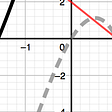## A Few Great Tips on How to Tackle the GMAT DS Questions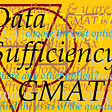## Natural Philosophy: Thinking as Rene Descartes Might## The Limits of Logic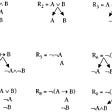## GMAT Algebra Problem — Parts — Hotdogs & Donuts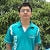## Re rooting the Tree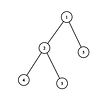## Divide and Conquer: Karatsuba Integer Multiplication